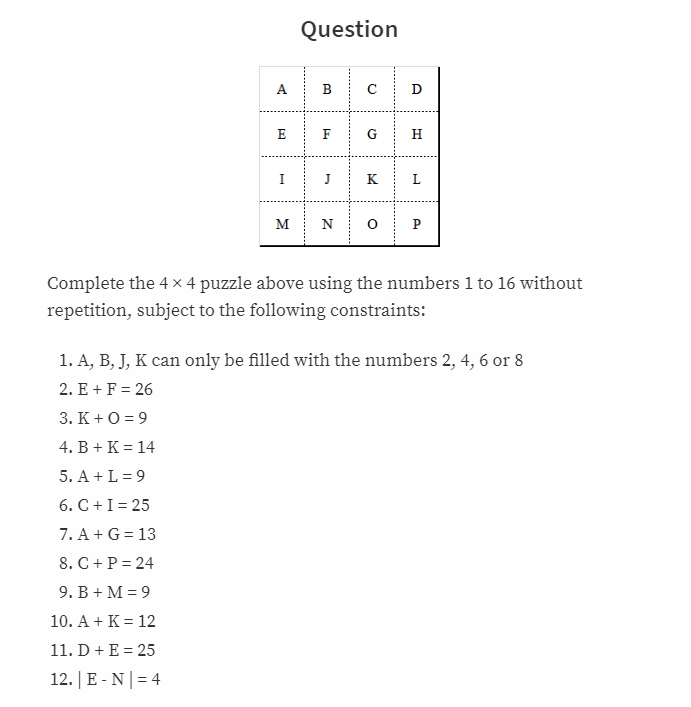# Bruteforce Solution to Mathematics × Programming Competition #5 暴力搜索[問題] 數學 × 程式編寫比賽 (第五回)steemCreated with Sketch.

in cn •  2 years ago  (edited)

@kenchung 第五次比赛 #steemstem, 题目主要是A到P 16个字母填到4X4的格子中，1-16只能各用一次，并且有12个条件。
Quesiton: https://steemit.com/contest/@kenchung/question-mathematics-programming-competition-5Solution:
16 integers, but the total combination is huge i.e. 16! (A has 16 possibilities, B has 15 possibilities and so on).

The good thing is that we have a few of restrictions such as A can only be 2, 4, 6 or 8 and etc. Therefore, we can loop just few variables and base on pre-known conditions to get the values of others.

The following JS code is ugly, which implements the bruteforce search, however it works.. Let me think of better ways to write it and I'd share with you in a few days time.

``````
//4,6,13,15,10,16,9,7,12,2,8,5,3,14,1,11

for (var a = 2; a <= 8; a += 2) {
var l = 9 - a;
var g = 13 - a;
var k = 12 - a;
if (k == a) {
continue;
}
if ((k != 2) && (k != 4) && (k != 6) && (k != 8)) {
continue;
}
var b = 14 - k;
if ((b == a) || (b == k)) {
continue;
}
if ((b != 2) && (b != 4) && (b != 6) && (b != 8)) {
continue;
}
var o = 9 - k;
var m = 9 - b;
for (var j = 2; j <= 8; j += 2) {
if ((j != a) && (j != b) && (j != k)) {
// c, d, e, f, h, i, n, p
// c + p = 24
// d + e = 25
// |e - n| = 4
// e + f = 26
// c + i = 25
console.log('a = ' + a);
console.log('b = ' + b);
console.log('g = ' + g);
console.log('k = ' + k);
console.log('o = ' + o);
console.log('m = ' + m);
console.log('j = ' + j);
console.log('l = ' + l);
for (var c = 1; c <= 16; c ++) {
if ((c != a) && (c != b) && (c != g) && (c != k) && (c != o) && (c != m) && (c != j) && (c != l)) {
var p = 24 - c;
if (c == p) continue;
if ((p < 1) || (p > 16)) continue;
var i = 25 - c;
if ((i < 1) || (i > 16)) continue;
for (d = 1; d <= 16; d ++) {
if ((d != c) && (d != a) && (d != b) && (d != g) && (d != k) && (d != o) && (d != m) && (d != j) && (d != l)) {
var e = 25 - d;
if ((e < 1) || (e > 16)) continue;
var f = 26 - e;
if ((f < 1) || (f > 16)) continue;
n = e + 4;
if ((n < 1) || (n > 16)) continue;
if (
(i == n) ||
(n == f) ||
(i == d) ||
(p == k) ||
(n == k) ||
(n == b) ||
(e == f) ||
(p == e) ||
(i == f) ||
(p == f) ||
(p == n) ||
(p == g) ||
(c == f)
) {
continue;
}
console.log("p = " + p);
console.log("i = " + i);
console.log("n = " + n);
console.log("e = " + e);
console.log("f = " + f);
console.log("c = " + c);
console.log("d = " + d);
}
}
}
}
}
}
}
``````

This outputs:

``````a = 4
b = 6
g = 9
k = 8
o = 1
m = 3
j = 2
l = 5
p = 11
i = 12
n = 14
e = 10
f = 16
c = 13
d = 15
[Finished in 0.7s]
``````

Wait, the h is not printed (in the 12 pre-known relations, h is not specified)... but you know what to do. ;)

h 值没有被打出来，因为这是唯一一个没有给定限制条件的变量，不过你应该知道怎么做了。

Originally published at https://steemit.com Thank you for reading my post, feel free to Follow, Upvote, Reply, ReSteem (repost) @justyy which motivates me to create more quality posts.

// Later, it will be reposted to my blogs: justyy.com, helloacm.com and codingforspeed.com 稍后同步到我的中文博客和英文计算机博客

# Recent Popular PostsTags: #cn #cn-programming #programming #steemstem

Sort Order:
·  2 years ago

Did you write this code?

·  2 years ago

yes, i know this is ugly, but i do that to win the contest.

·  2 years ago

I like math, interesting quiz :) idk but I'm not good at programming so I decided to look if I can get here system to solve it with gauss method :D well I just passed, programming is better anyway for finding solution and checking all 16! Possibilities

·  2 years ago

·  2 years ago

NB，菊大哥推理功力很强。

·  2 years ago

·  2 years ago

·  2 years ago

·  2 years ago

·  2 years ago

·  2 years ago

Nice!
Do you know Prolog? I solved this problem using it. The idea is the same as you did. Only all the loops to try new values for each variable are automatically handled by the Prolog engine 😼
You can see my solution here:
https://steemit.com/contest/@armandocat/mathematics-programming-competition-5-prolog-solution

·  2 years ago Reveal Comment